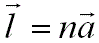Lattice Systems
- Practical Electron Microscopy and Database -
- An Online Book -

https://www.globalsino.com/EM/

 This book (Practical Electron Microscopy and Database) is a reference for TEM and SEM students, operators, engineers, technicians, managers, and researchers. ================================================================================= A lattice symmetry has the following basic characteristics:          i) Each lattice point must have identical surroundings, i.e., have the same environment.          ii) In a given direction, all lattice points must be separated by an identical distance, so-called lattice parameter. As shown in Figure 4545a, for an one-dimensional (1-D) lattice, a translation of l from one lattice point to another will present an “identical” lattice:-----------------------------  where,         n -- An integer.         l and a -- Vectors.Figure 4545a. One-dimensional (1-D) lattice. As shown in Figure 4545b, a two-dimensional (2-D) lattice has the following characteristics:         i) It has two non-collinear basis vectors (a and b). The interaxial angle γ determines the relationship between the two basis vectors.         ii) A translation of na + pb (e.g. 1a + 3b in Figure 4545b) from one lattice point to another must form an “identical” lattice structure. Here, n and p are  integers.Figure 4545b. Two-dimensional (2-D) lattice. As shown in Figure 4545c, a three-dimensional (3-D) lattice has the following characteristics:         i) It has 3 non-collinear basis vectors (a, b, and c) and 3 interaxial angles (α, β, and γ).         ii) All points can be determined by a series of vectors:----------------------------------Figure 4545c. Three-dimensional (3-D) lattice. Note that crystal lattices must exhibit a specific minimal amount of symmetry, and each crystal system has a certain symmetry. Table 4545 lists the relationship between three-dimensional crystal families, crystal systems, and lattice systems. Table 4545. Relationship between three-dimensional crystal families, crystal systems, and lattice systems.=================================================================================

The book author (Yougui Liao) welcomes your comments, suggestions, and corrections, please click here for submission. If you let book author know once you have cited this book, the brief information of your publication will appear on the “Times Cited” page.